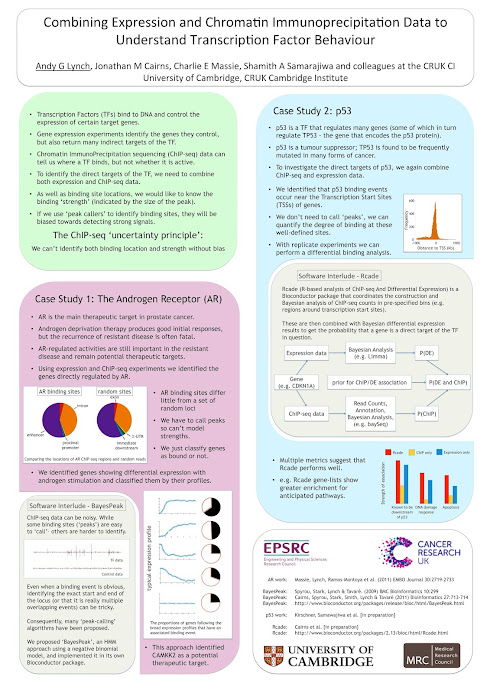Home‎ > ‎

Bits and Pieces

Poster presented at the International Biometric Society Channel Network Conference at St. Andrews

posted 5 Jul 2013, 06:58 by Andy Lynch

 Always tricky when competing with food and wine, but it was good to have the chance to chat to some people about this work.Drawing the mitochondrial genome in R with grid graphics

posted 26 May 2013, 04:31 by Andy Lynch

 Possibly I should be using circos plots for this, but I find them tricky to configure and I don't want to have to break the D-loop into two pieces because of a discontinuity at 0. Therefore I shall raid Paul Murrell's website for the bits of grid graphics technology that I require, MitoMap for the coordinates and try to do it all in R.library(grid)The key to this is the ability to draw a segment of a ring.plotsegment <- function(xc, yc, angle1,angle2,outer,inner,n=50, gp=gpar()) {  t1 <- seq(angle1, angle2, length=n)  x1 <- sin(t1)  y1 <- cos(t1)  xx <- xc + outer*x1  yy <- yc + outer*y1  t2 <- seq(angle1, angle2, length=n)  x2 <- sin(t2)  y2 <- cos(t2)  xxx <- xc + inner*x2  yyy <- yc + inner*y2  grid.path(c(xx, rev(xxx)), c(yy, rev(yyy)),            id=c(rep(1, each=2*n)),            gp=gp)}Then it is just a case of grabbing coordinates from MitoMapDloopStart<-16024DloopEnd<-576+16569tRNAStart<-c(577,1602,3230,4263,4329,             4402,5512,5587,5657,5761,5826,7446,             7518,8259,9991,10405,12138,12207,             12266,14674,15888,15956)tRNAEnd<-c(647,1670,3304,4331,4400,           4469,5579,5655,5729,5826,5891,7514,           7585,8364,10058,10469,12206,12265,           12336,14742,15953,16023)rRNAStart<-c(648,1671)rRNAEnd<-c(1601,3229)geneStart<-c(3307,4470,5904,7586,8366,             8527,9207,10059,10470,10760,12337,             14149,14747)geneEnd<-c(4262,5511,7445,8269,8572,           9207,9990,10404,10766,12137,14148,           14673,15887)I then just need to define the viewport...circlevp <- viewport(x=.1,                   y=.1,                   width=.8,                   height=.8,                   just=c("left", "bottom"),                   name="plotRegion")pushViewport(circlevp)...and get plotting.for(i in 1:13){  plotsegment(0.5,0.5,geneStart[i]*2*pi/16569,geneEnd[i]*2*pi/16569,.5,.4,gp=gpar(fill="red"))  }for(i in 1:22){  plotsegment(0.5,0.5,tRNAStart[i]*2*pi/16569,tRNAEnd[i]*2*pi/16569,.47,.43,gp=gpar(fill="blue"))}for(i in 1:2){  plotsegment(0.5,0.5,rRNAStart[i]*2*pi/16569,rRNAEnd[i]*2*pi/16569,.48,.42,gp=gpar(fill="purple"))}for(i in 1:1){  plotsegment(0.5,0.5,DloopEnd[i]*2*pi/16569,DloopStart[i]*2*pi/16569,.46,.44,gp=gpar(fill="brown"))}I can then add to the plot to get pictures such as this:Clearly there are still things to tidy up, such as the overlap of ND4L and ND4 (and the colour scheme), but this seems to be a useful starting point.

1-2 of 2